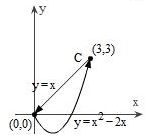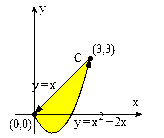# Use Green's Theorem to find the counterclockwise circulation and outward flux for the field F and...

## Question:

Use Green's Theorem to find the counterclockwise circulation and outward flux for the field {eq}\vec{F} {/eq} and curve {eq}C {/eq}.

{eq}\vec{F}=15x^3y^2\,\vec{i}+\frac{15}{2}x^{4}y\,\vec{j} {/eq}## Green's Theorem; Iterated Integrals:

By means of Green's Theorem, we can evaluate the line integral around a closed curve, by means of an iterated integral in the plane. Namely, for a smooth vector field

{eq}\vec{F}(x,y)=P(x,y)\,\vec{i}+Q(x,y)\,\vec{j} {/eq}

and a closed curve {eq}C {/eq} oriented positively then,

{eq}\int_{C^+} \vec{F}\cdot d\vec{r} = \iint_D (Q_x-P_y)\,dx\,dy, {/eq}

where {eq}D {/eq} is the region in the plane enclosed by the bounding curve {eq}C {/eq}.

We notice that in this problem, the curve {eq}C {/eq} consists of two smooth curves, forming a closed path.

## Answer and Explanation:

The diagram below shows the yellow the shaded region {eq}D, {/eq} enclosed by a portion of the parabola {eq}y=x^2-2x {/eq} and the segment connecting the point {eq}(3,3) {/eq} to the origin.To evaluate the line integral we have:

{eq}\begin{align*} I&=\int_C 15x^3y^2\, dx+\frac{15}{2}x^{4}y \, dy\\ &= \int_{C^+} \vec{F}\cdot d\vec{r} \end{align*} {/eq}

which allows us to use Green's Theorem directly for the closed path {eq}C, {/eq} and we cab say that for the smooth vector field,

{eq}\vec{F}(x,y)= P(x,y)\vec{i} + \ Q(x,y)\vec{j}= 15x^3y^2\,\vec{i}+\frac{15}{2}x^{4}y\,\vec{j} {/eq}

and the closed path {eq}C {/eq} (which is oriented positively) then,

{eq}I=\int_{C} \vec{F}\cdot d\vec{r} = \iint_D (Q_x-P_y)\,dx\,dy, {/eq}

where {eq}D {/eq} is the region in the plane enclosed by the curve {eq}C {/eq}.

Now we can evaluate {eq}I {/eq}:

{eq}\begin{align*} I&=\int_{C} 15x^3y^2\,dx+\frac{15}{2}x^{4}y \, dy,\\ &\text{use Green's Theorem}\\ &=\iint_D (Q_x-P_y)\,dx\,dy\\ &=\iint_D \left(30x^3y-30x^3y\right)\,dx\,dy\\ &=\iint_D 0\;dx\,dy\\ &\text{the integrand is equal to zero}\\ &\text{so the integral is equal to }0,\\ &I=0. \end{align*} {/eq}

We notice that the integrand of the iterated integral is equal to zero, so we have:

{eq}I= \int_{C} 15x^3y^2\, dx+\frac{15}{2}x^{4}y \, dy=0. {/eq}

#### Learn more about this topic:Double Integrals & Evaluation by Iterated Integrals

from GRE Math: Study Guide & Test Prep

Chapter 15 / Lesson 4
401